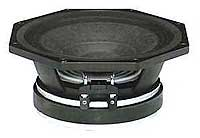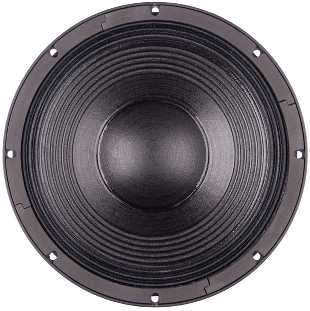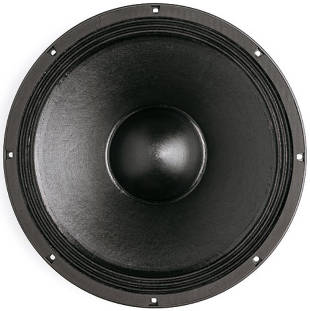# B&C

Founded in 1946, B&C Speakers is a professional loudspeaker transducer manufacturers in Italy.

In addition to designing and distributing components under the B&C brand name, they also supply OEM components to many of the top professional audio brands in the market today.

## Wooferrecommended cabinet 1:
closed cabinet with 1,1 L volume
from 238/150 Hz (-3dB/-8dB)

recommended cabinet 2:
4,2 L volume bass reflex cabinet
with HP70 reflex tube, 12 cm long.
from 115/94 Hz (-3dB/-8dB).

## B&C 6 MD 38

order no. bc-6md38

EUR 119,50excl. VAT: € 100.42 / \$ 108.45

17 cm midrange driver with high quality cone.

• power handling (continuous/programme) = 120/240 W
• frequency range = 150-6000 Hz
• resonance frequency fs = 130 Hz
• impedance R = 8 Ohm
• sound pressure level SPL = 96 dB (2,83V; 1m)
• DC resistance Re = 5,7 Ohm
• force factor BL = 10,5 N/A
• voice coil inductance L = 0,25 mH
• effective piston radiating area Sd = 132 cm2
• effective mechanical mass incl. air load mms = 12 g
• equivalent volume of compliance Vas = 3 l
• total Q factor Qts = 0,43 (Qms=3,7, Qes=0,49)
• voice coil diameter = 38 mm
• maximum peak linear excursion vibration xlin = +/- 2 mm
• mounting diameter d = 145 mm
• overall diameter d = 187 mm
• mounting depth (not countersunk) t = 82 mm
• mass m = 2,2 kg
PDF data sheetrecommended cabinet 1:
closed cabinet with 0,68 L volume
from 358/226 Hz (-3dB/-8dB)

recommended cabinet 2:
1,2 L volume bass reflex cabinet
with HP70 reflex tube, 22 cm long.
from 241/178 Hz (-3dB/-8dB).

## B&C 8 PE 21

order no. bc-8pe21

EUR 147,70excl. VAT: € 124.12 / \$ 134.05

21 cm midrange driver with high quality cone.

• power handling (continuous/programme) = 200/400 W
• frequency range = 90-5000 Hz
• resonance frequency fs = 87 Hz
• impedance R = 8 Ohm
• sound pressure level SPL = 98 dB (2,83V; 1m)
• DC resistance Re = 5,6 Ohm
• force factor BL = 16,6 N/A
• voice coil inductance L = 0,5 mH
• effective piston radiating area Sd = 220 cm2
• effective mechanical mass incl. air load mms = 18 g
• equivalent volume of compliance Vas = 13 l
• total Q factor Qts = 0,19 (Qms=3,8, Qes=0,2)
• voice coil diameter = 50 mm
• maximum peak linear excursion vibration xlin = +/- 1 mm
• mounting diameter d = 187 mm
• overall diameter d = 225 mm
• mounting depth (not countersunk) t = 91 mm
• mass m = 4,2 kg
PDF data sheetrecommended cabinet 1:
closed cabinet with 2,4 L volume
from 177/112 Hz (-3dB/-8dB)

recommended cabinet 2:
7,7 L volume bass reflex cabinet
with HP70 reflex tube, 12 cm long.
from 94/73 Hz (-3dB/-8dB).

## B&C 8 PS 21

order no. bc-8ps21

EUR 144,40excl. VAT: € 121.34 / \$ 131.05

21 cm bass driver with high quality cone.

• power handling (continuous/programme) = 200/400 W
• frequency range = 70-3000 Hz
• resonance frequency fs = 73 Hz
• impedance R = 8 Ohm
• sound pressure level SPL = 94 dB (2,83V; 1m)
• DC resistance Re = 5,4 Ohm
• force factor BL = 12,6 N/A
• voice coil inductance L = 0,5 mH
• effective piston radiating area Sd = 220 cm2
• effective mechanical mass incl. air load mms = 23 g
• equivalent volume of compliance Vas = 14 l
• total Q factor Qts = 0,33 (Qms=4,2, Qes=0,36)
• voice coil diameter = 50 mm
• maximum peak linear excursion vibration xlin = +/- 5 mm
• mounting diameter d = 187 mm
• overall diameter d = 225 mm
• mounting depth (not countersunk) t = 91 mm
• mass m = 3,7 kg
PDF data sheetopen a bigger photoopen a bigger photo

recommended cabinet 1:
closed cabinet with 5,2 L volume
from 144/91 Hz (-3dB/-8dB)

recommended cabinet 2:
15 L volume bass reflex cabinet
with HP100 reflex tube, 21 cm long.
from 79/61 Hz (-3dB/-8dB).

## B&C 10 PS 26

order no. bc-10ps26

EUR 224,10excl. VAT: € 188.32 / \$ 203.38

25 cm bass driver with high quality cone.

• power handling (continuous/programme) = 350/700 W
• frequency range = 55-4000 Hz
• resonance frequency fs = 54 Hz
• impedance R = 8 Ohm
• sound pressure level SPL = 96 dB (2,83V; 1m)
• DC resistance Re = 5,8 Ohm
• force factor BL = 14,1 N/A
• voice coil inductance L = 1,2 mH
• effective piston radiating area Sd = 320 cm2
• effective mechanical mass incl. air load mms = 33 g
• equivalent volume of compliance Vas = 38 l
• total Q factor Qts = 0,3 (Qms=2,9, Qes=0,33)
• voice coil diameter = 76 mm
• maximum peak linear excursion vibration xlin = +/- 5,5 mm
• mounting diameter d = 230 mm
• overall diameter d = 262 mm
• mounting depth (not countersunk) t = 123 mm
• mass m = 6 kg
PDF data sheetrecommended cabinet 1:
closed cabinet with 1,3 L volume
from 285/180 Hz (-3dB/-8dB)

recommended cabinet 2:
2,6 L volume bass reflex cabinet
with HP70 reflex tube, 15 cm long.
from 183/136 Hz (-3dB/-8dB).

## B&C 10 MD 26

order no. bc-10md26

EUR 224,10excl. VAT: € 188.32 / \$ 203.38

25 cm midrange driver with high quality cone.

• power handling (continuous/programme) = 350/700 W
• frequency range = 80-4000 Hz
• resonance frequency fs = 76 Hz
• impedance R = 8 Ohm
• sound pressure level SPL = 100 dB (2,83V; 1m)
• DC resistance Re = 5,8 Ohm
• force factor BL = 19,6 N/A
• voice coil inductance L = 1,45 mH
• effective piston radiating area Sd = 320 cm2
• effective mechanical mass incl. air load mms = 31 g
• equivalent volume of compliance Vas = 20 l
• total Q factor Qts = 0,21 (Qms=4,8, Qes=0,22)
• voice coil diameter = 76 mm
• maximum peak linear excursion vibration xlin = +/- 1,5 mm
• mounting diameter d = 230 mm
• overall diameter d = 262 mm
• mounting depth (not countersunk) t = 124 mm
PDF data sheetrecommended cabinet 1:
closed cabinet with 4,7 L volume
from 157/99 Hz (-3dB/-8dB)

recommended cabinet 2:
14,4 L volume bass reflex cabinet
with HP100 reflex tube, 18 cm long.
from 86/66 Hz (-3dB/-8dB).

## B&C Neodym 10 HPL 64

order no. bc-10hpl64

EUR 187,60excl. VAT: € 157.65 / \$ 170.26

25 cm bass driver with high quality cone.

• power handling (continuous/programme) = 200/400 W
• frequency range = 60-4000 Hz
• resonance frequency fs = 61 Hz
• impedance R = 8 Ohm
• sound pressure level SPL = 98,5 dB (2,83V; 1m)
• DC resistance Re = 5,4 Ohm
• force factor BL = 15 N/A
• voice coil inductance L = 15 mH
• effective piston radiating area Sd = 320 cm2
• effective mechanical mass incl. air load mms = 29 g
• equivalent volume of compliance Vas = 32 l
• total Q factor Qts = 0,31 (Qms=4,5, Qes=0,33)
• voice coil diameter = 64 mm
• maximum peak linear excursion vibration xlin = +/- 4 mm
• mounting diameter d = 230 mm
• overall diameter d = 261 mm
• mounting depth (not countersunk) t = 122 mm
PDF data sheetrecommended cabinet 1:
closed cabinet with 4,7 L volume
from 221/140 Hz (-3dB/-8dB)

recommended cabinet 2:
8 L volume bass reflex cabinet
with HP100 reflex tube, 17 cm long.
from 152/113 Hz (-3dB/-8dB).

## B&C 12 PE 32

order no. bc-12pe32

EUR 217,50excl. VAT: € 182.77 / \$ 197.39

30 cm bass driver with high quality cone.

• power handling (continuous/programme) = 250/500 W
• frequency range = 50-4000 Hz
• resonance frequency fs = 51 Hz
• impedance R = 8 Ohm
• sound pressure level SPL = 101,5 dB (2,83V; 1m)
• DC resistance Re = 5,8 Ohm
• force factor BL = 19,1 N/A
• voice coil inductance L = 1,4 mH
• effective piston radiating area Sd = 530 cm2
• effective mechanical mass incl. air load mms = 37 g
• equivalent volume of compliance Vas = 101 l
• total Q factor Qts = 0,19 (Qms=7,6, Qes=0,19)
• voice coil diameter = 65 mm
• maximum peak linear excursion vibration xlin = +/- 2,8 mm
• mounting diameter d = 282 mm
• overall diameter d = 316 mm
• mounting depth (not countersunk) t = 135 mm
PDF data sheetrecommended cabinet 1:
closed cabinet with 3,7 L volume
from 214/135 Hz (-3dB/-8dB)

recommended cabinet 2:
6,7 L volume bass reflex cabinet
with HP100 reflex tube, 23 cm long.
from 144/107 Hz (-3dB/-8dB).

## B&C 12 MH 32

order no. bc-12mh32

EUR 255,60excl. VAT: € 214.79 / \$ 231.97

30 cm bass driver with high quality cone.

• power handling (continuous/programme) = 400/800 W
• frequency range = 50-3000 Hz
• resonance frequency fs = 52 Hz
• impedance R = 8 Ohm
• sound pressure level SPL = 101 dB (2,83V; 1m)
• DC resistance Re = 5,4 Ohm
• force factor BL = 21,3 N/A
• voice coil inductance L = 1,4 mH
• effective piston radiating area Sd = 530 cm2
• effective mechanical mass incl. air load mms = 50 g
• equivalent volume of compliance Vas = 71 l
• total Q factor Qts = 0,19 (Qms=6,3, Qes=0,2)
• voice coil diameter = 76 mm
• maximum peak linear excursion vibration xlin = +/- 3,5 mm
• mounting diameter d = 282 mm
• overall diameter d = 316 mm
• mounting depth (not countersunk) t = 134 mm
PDF data sheetopen a bigger photoopen a bigger photo

recommended cabinet 1:
closed cabinet with 5,1 L volume
from 129/82 Hz (-3dB/-8dB)

recommended cabinet 2:
13,6 L volume bass reflex cabinet
with HP100 reflex tube, 33 cm long.
from 75/57 Hz (-3dB/-8dB).

## B&C 12 PS 100

order no. bc-12ps100

EUR 308,80excl. VAT: € 259.50 / \$ 280.26

30 cm bass driver with high quality cone.

• power handling (continuous/programme) = 700/1400 W
• frequency range = 45-1000 Hz
• resonance frequency fs = 44 Hz
• impedance R = 8 Ohm
• sound pressure level SPL = 93 dB (2,83V; 1m)
• DC resistance Re = 5,3 Ohm
• force factor BL = 22,5 N/A
• voice coil inductance L = 2 mH
• effective piston radiating area Sd = 531 cm2
• effective mechanical mass incl. air load mms = 106 g
• equivalent volume of compliance Vas = 47 l
• total Q factor Qts = 0,27 (Qms=3,9, Qes=0,29)
• voice coil diameter = 100 mm
• maximum peak linear excursion vibration xlin = +/- 8 mm
• mounting diameter d = 281 mm
• overall diameter d = 319 mm
• mounting depth (not countersunk) t = 118 mm
PDF data sheetrecommended cabinet 1:
closed cabinet with 13,6 L volume
from 130/82 Hz (-3dB/-8dB)

recommended cabinet 2:
42 L volume bass reflex cabinet
with 2x HP100 reflex tube, 14 cm long.
from 70/54 Hz (-3dB/-8dB).

## B&C Neodym 12 HPL 64

order no. bc-12hpl64

EUR 214,10excl. VAT: € 179.92 / \$ 194.31

30 cm bass driver with high quality cone.

• power handling (continuous/programme) = 200/400 W
• frequency range = 50-4000 Hz
• resonance frequency fs = 52 Hz
• impedance R = 8 Ohm
• sound pressure level SPL = 100 dB (2,83V; 1m)
• DC resistance Re = 5,8 Ohm
• force factor BL = 14,9 N/A
• voice coil inductance L = 1,1 mH
• effective piston radiating area Sd = 522 cm2
• effective mechanical mass incl. air load mms = 41,5 g
• equivalent volume of compliance Vas = 85 l
• total Q factor Qts = 0,32 (Qms=3,3, Qes=0,35)
• voice coil diameter = 64 mm
• maximum peak linear excursion vibration xlin = +/- 4 mm
• mounting diameter d = 282 mm
• overall diameter d = 315 mm
• mounting depth (not countersunk) t = 144 mm
PDF data sheetrecommended cabinet 1:
closed cabinet with 7 L volume
from 168/106 Hz (-3dB/-8dB)

recommended cabinet 2:
16 L volume bass reflex cabinet
with HP100 reflex tube, 14 cm long.
from 104/78 Hz (-3dB/-8dB).

## B&C Neodym 12 HPL 76

order no. bc-12hpl76

EUR 270,60excl. VAT: € 227.39 / \$ 245.59

30 cm bass driver with high quality cone.

• power handling (continuous/programme) = 350/700 W
• frequency range = 50-3500 Hz
• resonance frequency fs = 49 Hz
• impedance R = 8 Ohm
• sound pressure level SPL = 99 dB (2,83V; 1m)
• DC resistance Re = 5,7 Ohm
• force factor BL = 17,3 N/A
• voice coil inductance L = 1,15 mH
• effective piston radiating area Sd = 531 cm2
• effective mechanical mass incl. air load mms = 43 g
• equivalent volume of compliance Vas = 91 l
• total Q factor Qts = 0,24 (Qms=2,5, Qes=0,26)
• voice coil diameter = 76 mm
• maximum peak linear excursion vibration xlin = +/- 4 mm
• mounting diameter d = 282 mm
• overall diameter d = 315 mm
• mounting depth (not countersunk) t = 144 mm
PDF data sheet

## 15 inch wooferopen a bigger photoopen a bigger photo

recommended cabinet 1:
closed cabinet with 8,9 L volume
from 145/92 Hz (-3dB/-8dB)

recommended cabinet 2:
17 L volume bass reflex cabinet
with HP100 reflex tube, 19 cm long.
from 95/71 Hz (-3dB/-8dB).

## B&C 15 PL 100

order no. bc-15pl100

EUR 322,00excl. VAT: € 270.59 / \$ 292.24

38 cm bass driver with high quality cone.

• power handling (continuous/programme) = 700/1400 W
• frequency range = 35-2000 Hz
• resonance frequency fs = 37 Hz
• impedance R = 8 Ohm
• sound pressure level SPL = 97 dB (2,83V; 1m)
• DC resistance Re = 5,3 Ohm
• force factor BL = 26,8 N/A
• voice coil inductance L = 1,7 mH
• effective piston radiating area Sd = 855 cm2
• effective mechanical mass incl. air load mms = 121 g
• equivalent volume of compliance Vas = 154 l
• total Q factor Qts = 0,2 (Qms=6,6, Qes=0,21)
• voice coil diameter = 100 mm
• maximum peak linear excursion vibration xlin = +/- 7 mm
• mounting diameter d = 354 mm
• overall diameter d = 393 mm
• mounting depth (not countersunk) t = 173 mm
PDF data sheet

## 18 inch wooferrecommended cabinet 1:
closed cabinet with 24 L volume
from 111/70 Hz (-3dB/-8dB)

recommended cabinet 2:
67 L volume bass reflex cabinet
with 4x HP100 reflex tube, 34 cm long.
from 63/48 Hz (-3dB/-8dB).

## B&C 18 PS 76

order no. bc-18ps76

EUR 303,80excl. VAT: € 255.29 / \$ 275.72

44 cm bass driver with high quality cone.

• power handling (continuous/programme) = 600/1200 W
• frequency range = 40-2000 Hz
• resonance frequency fs = 39 Hz
• impedance R = 8 Ohm
• sound pressure level SPL = 99 dB (2,83V; 1m)
• DC resistance Re = 5,3 Ohm
• force factor BL = 25,8 N/A
• voice coil inductance L = 1,9 mH
• effective piston radiating area Sd = 1210 cm2
• effective mechanical mass incl. air load mms = 149 g
• equivalent volume of compliance Vas = 207 l
• total Q factor Qts = 0,28 (Qms=6,1, Qes=0,29)
• voice coil diameter = 76 mm
• maximum peak linear excursion vibration xlin = +/- 7 mm
• mounting diameter d = 422 mm
• overall diameter d = 460 mm
• mounting depth (not countersunk) t = 202 mm
PDF data sheet

The cabinet volume calculations are executed considering an external resistance of 0.3 Ohm. We are using, if no other data is known or available, the information supplied by the manufacturer.

Please note: the frequency response doesn't say much about the cut-off frequency inside the cabinet! Therefore, we mostly give you 2 examples with dimensioning considerations and an indication, how low the loudspeaker will reproduce.

Home